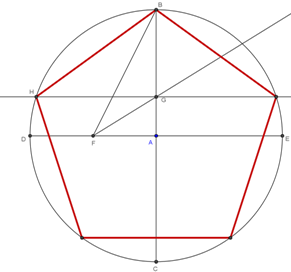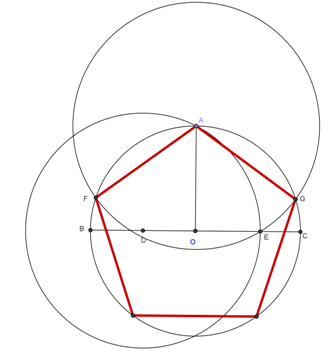## Method 1

• Draw circle centre A
• Draw perpendicular diameters BC and DE
• label F
• Construct line BF
• Construct bisector of angle AFB
• Label G - the intersection of bisector and AB
• Draw parallel to CD through G
• Label H intersection of parallel with circle
• BH is one side of pentagon
• complete pentagon## Method 2

• Draw circle centre O, radius OA
• Construct 2 radii perpendicular to OA
• label OB and OC
• Construct D, the midpoint of OB
• Draw circle, centre D, radius DA
• label E, the intersection with OC
• Draw circle, centre A, radius AE
• label F and G, the intersection with the original circle
• AF and AG are two sides of the pentagon
• complete pentagon